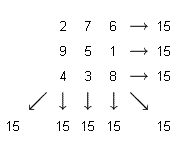Problems

Magic square

Magic square - a square table, filled n2 numbers so that the sum of the numbers in each row, each column and both diagonals of the same. Sum of the numbers in each row, column and diagonal, is called the magic constant. Check or specify the square is magical.Input

The first line contains the number n(1n100).Next is the n rows of n numbers each. All of the square - the natural numbers not exceeding 32000.

Output

If the specified array is magic - the magic of its output constant, otherwise the output -1.

Time limit 1 second
Memory limit 64 MiB
Input example #1
3
2 7 6
9 5 1
4 3 8
Output example #1
15